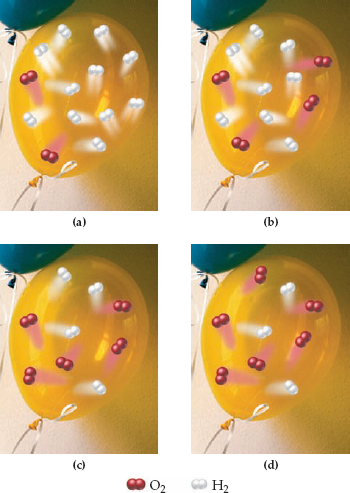×
Log in to StudySoup
Get Full Access to Introductory Chemistry - 5 Edition - Chapter 8 - Problem 99p
Join StudySoup for FREE
Get Full Access to Introductory Chemistry - 5 Edition - Chapter 8 - Problem 99p

Already have an account? Login here
×
Reset your password

# Solved: A loud classroom demonstration involves igniting a hydrogen-filled balloon. TheISBN: 9780321910295 34

## Solution for problem 99P Chapter 8

Introductory Chemistry | 5th Edition

• Textbook Solutions
• 2901 Step-by-step solutions solved by professors and subject experts
• Get 24/7 help from StudySoup virtual teaching assistantsIntroductory Chemistry | 5th Edition

4 5 1 425 Reviews
11
2
Problem 99P

PROBLEM 99P

A loud classroom demonstration involves igniting a hydrogen-filled balloon. The hydrogen within the balloon reacts explosively with oxygen in the air to form water according to this reaction:

2 H2(g) + O2(g) → 2 H2O(g)

If the balloon is filled with a mixture of hydrogen and oxygen, the explosion is even louder than if the balloon is filled with only hydrogen; the intensity of the explosion depends on the relative amounts of oxygen and hydrogen within the balloon. Consider the molecular views representing different amounts of hydrogen and oxygen in four different balloons. Based on the balanced chemical equation, which balloon will make the loudest explosion?Step-by-Step Solution:

Problem 99P

A loud classroom demonstration involves igniting a hydrogen-filled balloon. The hydrogen within the balloon reacts explosively with oxygen in the air to form water according to this reaction:If the balloon is filled with a mixture of hydrogen and oxygen, the explosion is even louder than if the balloon is filled with only hydrogen; the intensity of the explosion depends on the relative amounts of oxygen and hydrogen within the balloon. Consider the molecular views representing different amounts of hydrogen and oxygen in four different balloons. Based on the balanced chemical equation, which balloon will make the loudest explosion?Step by Step Solution

Step 1 of 2

The balanced chemical equation is as follows.From the balanced chemical equation, two moles of hydrogen reacts with one mole of oxygen to form two moles of water.

Step 2 of 2

##### ISBN: 9780321910295

Introductory Chemistry was written by and is associated to the ISBN: 9780321910295. The answer to “A loud classroom demonstration involves igniting a hydrogen-filled balloon. The hydrogen within the balloon reacts explosively with oxygen in the air to form water according to this reaction:2 H2(g) + O2(g) ? 2 H2O(g)If the balloon is filled with a mixture of hydrogen and oxygen, the explosion is even louder than if the balloon is filled with only hydrogen; the intensity of the explosion depends on the relative amounts of oxygen and hydrogen within the balloon. Consider the molecular views representing different amounts of hydrogen and oxygen in four different balloons. Based on the balanced chemical equation, which balloon will make the loudest explosion?” is broken down into a number of easy to follow steps, and 104 words. This full solution covers the following key subjects: hydrogen, balloon, oxygen, explosion, filled. This expansive textbook survival guide covers 19 chapters, and 2046 solutions. Since the solution to 99P from 8 chapter was answered, more than 3229 students have viewed the full step-by-step answer. This textbook survival guide was created for the textbook: Introductory Chemistry, edition: 5. The full step-by-step solution to problem: 99P from chapter: 8 was answered by , our top Chemistry solution expert on 05/06/17, 06:45PM.

Unlock Textbook Solution

Enter your email below to unlock your verified solution to:

Solved: A loud classroom demonstration involves igniting a hydrogen-filled balloon. The